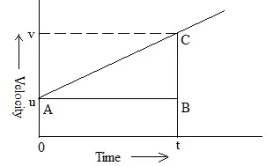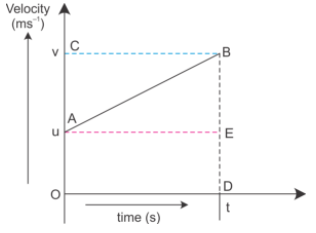# With the help of graph, derive the relation between:a. velocity timeb. position timec. position velocity (derive three equation of motion)

Velocity-Time

A car having initial velocity u is being subjected to a uniform acceleration a for a time period of t, after the time t the final velocity of the car is v.

Now we need to find the relation between v,u,a,t graphically.Initial velocity u at A = OA

Final velocity is V

Slope of velocity time graph is equal to acceleration a.

$a\ =\ \frac{v\ -\ u}{t\ -\ 0}$

$\Longrightarrow \ v\ =\ u\ +\ at$

Position -Time

Consider the linear motion of a body with an initial velocity u. Let the body accelerate uniformly and acquire a final velocity v after time t. The velocity–time graph is a straight line AB as shown below.At t = 0, initial velocity = u = OA

At t = t, final velocity = v = OC

The distance S traveled in time t = area of the trapezium OABD

$s\ =\ \frac{1}{2} \ \times \ ( OA\ +\ DB) \ \times \ OD$

$s\ =\ \frac{1}{2} \ \times \ ( u\ +\ v) \ \times \ t$

Since v = u + at,

$s\ =\ \frac{1}{2} \ \times \ ( u+\ u\ +\ at) \ \times \ t$

$s\ =\ ut\ +\ \frac{1}{2} at^{2}$

Velocity- Position

From, velocity time relation

$t\ =\ \frac{v\ -\ u}{a}$

substituting the value of 't' in equation $s\ =\ \frac{1}{2} \ \times \ ( u\ +\ v) \ \times \ t$

we get,

$s\ =\ \left(\frac{v\ +\ u}{2}\right) \ \times \ \left(\frac{v\ -\ u}{2}\right)$

=> v²=u²+2as

Updated on: 10-Oct-2022

29 Views